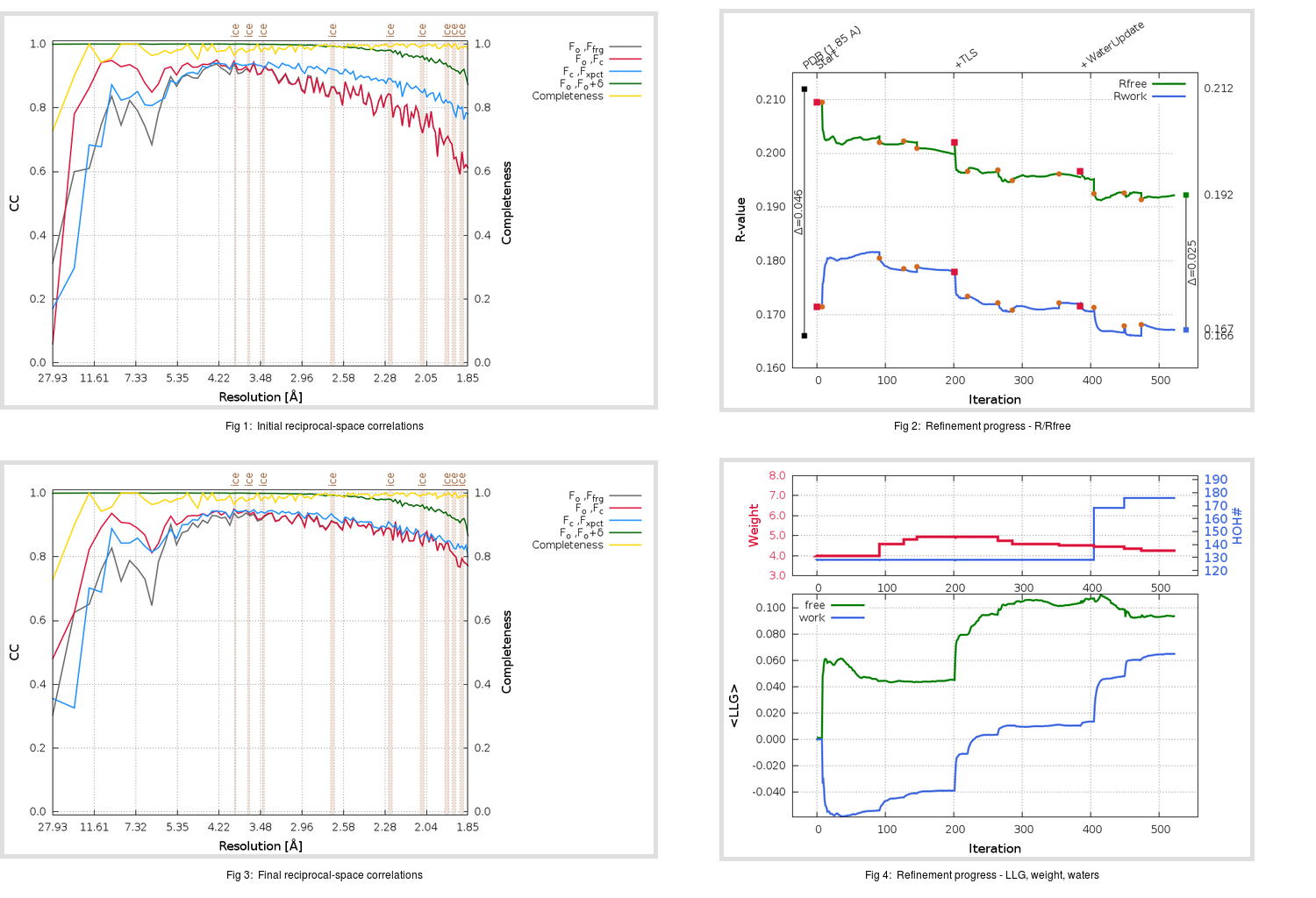Content:

```    Diffraction limits & principal axes of ellipsoid fitted to diffraction cut-off surface:
1.816         0.8792   0.0000   0.4765       0.998 a* + 0.063 c*
1.829         0.0000   1.0000   0.0000       b*
1.781        -0.4765   0.0000   0.8792      -0.699 a* + 0.716 c*
```

## Deposited

` `
 Date deposited Date data collection Resolution R, Rfree 20170725 20160625 1.85 0.1630 0.2120

Molprobity (CCP4 7.0 version) summary:

```Ramachandran outliers =   0.00 %
favored =  99.00 %
Rotamer outliers      =   0.42 %
C-beta deviations     =     0
Clashscore            =   2.24
RMS(bonds)            =   0.0107
RMS(angles)           =   1.35
MolProbity score      =   1.00
Resolution            =   1.85
R-work                =   0.1630
R-free                =   0.2120
```

```Number of waters      =   128

<B> (all atoms) =   28.45 ( sd =   11.11 ) for       2443 non-hydrogen atoms
<B>   (protein) =   28.21 ( sd =   11.16 ) for       2255 non-hydrogen atoms
<B>     (water) =   32.82 ( sd =    7.82 ) for        128 non-hydrogen atoms
<B>    (others) =   27.39 ( sd =   14.14 ) for         60 non-hydrogen atoms

B min/max       (all non-hydrogen atoms) =   10.84 /   80.38
B min/max   (protein non-hydrogen atoms) =   10.84 /   80.38
B min/max     (water non-hydrogen atoms) =   17.32 /   52.30
B min/max     (other non-hydrogen atoms) =   19.71 /   39.48
```

## BUSTER (re-)refinement

` `

Molprobity (CCP4 7.0 version) summary:

```Ramachandran outliers =   0.00 %
favored =  99.00 %
Rotamer outliers      =   0.42 %
C-beta deviations     =     0
Clashscore            =   0.90
RMS(bonds)            =   0.0110
RMS(angles)           =   1.56
MolProbity score      =   0.77
Resolution            =   1.85
R-work                =   0.1673
R-free                =   0.1922
```

```Number of waters      =   176

<B> (all atoms) =   28.84 ( sd =    9.58 ) for       2491 non-hydrogen atoms
<B>   (protein) =   28.07 ( sd =    8.86 ) for       2255 non-hydrogen atoms
<B>     (water) =   39.18 ( sd =   10.93 ) for        176 non-hydrogen atoms
<B>    (others) =   26.12 ( sd =   13.32 ) for         60 non-hydrogen atoms

B min/max       (all non-hydrogen atoms) =   13.85 /   83.87
B min/max   (protein non-hydrogen atoms) =   13.85 /   56.13
B min/max     (water non-hydrogen atoms) =   18.31 /   83.87
B min/max     (other non-hydrogen atoms) =   22.39 /   33.21
```

Refinement progression:Results:

` `
 File Remark 5WKL_aB_refine.01_03_refine.pdb.gz exact refinement commands are in header 5WKL_aB_refine.01_03_refine.mtz.gz including original deposited data and several re-refinement map coefficients 5WKL_aB_refine.01_03_BUSTER_model.cif.gz including any non-standard compound restraints 5WKL_aB_refine.01_03_BUSTER_refln.cif.gz